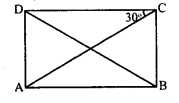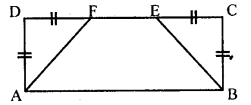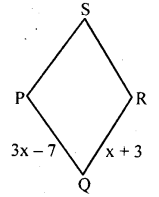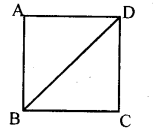# KSEEB Solutions for Class 8 Maths Chapter 15 Quadrilaterals Ex 15.4

In this chapter, we provide KSEEB SSLC Class 8 Maths Chapter 15 Quadrilaterals Ex 15.4 for English medium students, Which will very helpful for every student in their exams. Students can download the latest KSEEB SSLC Class 8 Maths Chapter 15 Quadrilaterals Ex 15.4 pdf, free KSEEB SSLC Class 8 Maths Chapter 15 Quadrilaterals Ex 15.4 pdf download. Now you will get step by step solution to each question.

### Karnataka State Syllabus Class 8 Maths Chapter 15 Quadrilaterals Ex 15.4

Question 1.
The sides of a rectangle are in the ratio 2: 1. The perimeter is 30 cm. Calculate the measure of all the sides.
The ratio of the sides is 2 : 1
Let the sides be 2x and x
Perimeter = 30
2x + x + 2x + x = 30
6x = 30
x = 36 = 5 cm
2x = 2 x 5 = 10cm
∴ The sides aer 10 cm, 5 cm, 10 cm, 5 cm.

Question 2.
In the adjacent rectangle ABCD ∠OCD = 30° . Calculate ∠BOC. What type of triangle is BOC.∠BCD = 90° [Angle of a rectangle]
∠OCD + ∠OCB = 90°
30 + ∠QCB = 90°
∠OCB = 90 – 30
∠OCB = 60°
∠OCB = ∠OBC = 60°
OC = OB [Diagonals of a rectangle bisect each other]
∴ ∠BOC = 180 – (60 + 60) = 180 – 120
∠BOC = 60°
In Δ BOC, ∠BOC = ∠OBC = ∠OCB = 60° & equiangular triangle
∴ BOC is an equiangular triangle.

Question 3.
All the rectangles are parallelograms but all the parallelograms are not rectangles. Justify this statement.
A rectangle has all the properties of a parallelogram, therefore, all rectangles are parallelograms. No angle of a Parallelogram is a right angle but all angles of a rectangle are right angles.
∴ All parallelograms are not rectangular.

Question 4.
The side of a rectangular park are in the ratio 4 : 3. If the area is 1728 m find the cost of fencing it at the rate of Rs . 2.50/m
The ratio of the sides = 4 : 3
Let the sides be 4x and 3x
Area = 1728
l × b = 1728
4x × 3x = 1728
12x2 = 1728
x2 = 121728
x2 = 144
x = √144 = 12m
4x = 4 × 12 = 48m
3x = 3x × 12 = 36m
Perimeter = 4x + 3x + 4x + 3x
= 48 + 36 + 48 + 36
= 168m
The cost of fencing 1m is Rs 2.50
The cost of fencing 168m = 168 × 250 = Rs. 420.

Question 5.
A rectangular yard contains two flower beds in the shape of congruent isosceles right triangles. The remaining portion is a yard of a trapezoidal shape whose parallel sides have length 15m and 25m. What fraction of the yard is occupied by the flower bed?In the fig AB = 25 m and FE = 15 m
∴ DF + EC = 25 – 15 = 10m
But DF = EC
∴DF = EC = 5m also AD = BC = 5m
Area of rectangle ∆BCD = AB x BC = 1 x b = 25 x 5 = 125 m2
Area of ∆ADF = 12 base × height
= 12 5 × 5 = 252m2
Area of ∆BCE = 252 m2
Sum of Area of the two triangles
∴ 15 of the field is occupied by the flower bed.

Question 6.
In a rhombus ABCD  ∠C = 70°. Find the other angles of the rhombus.
∠A = ∠C = 70° [Opposite angles]
∠A +∠B = 180° [Adjacent angles of a rhombus]
70 + ∠B = 180°
∠B = 180 – 70
∠B = 110°
∠B = ∠D = 110° [Opposite angles are equal].
Angles are 70°, 110°, 70° & 110°

Question 7.
In a rhombus PQRS, if PQ = 3x – 7 and QR = x + 3 find PS.PQ = QR [Sides of a rhombus]
3x – 7 = x + 3
3x – x = 3 + 7
2x = 10
102
x = 5
PQ = 3x – 7 = 3 × 5 – 7 = 15 – 7
PQ = 8 cm
∴ SP = 8

Question 8.
Rhombus is a parallelogram. Justify.
In a rhombus opposite sides are equal and parallel to each other therefore it is a parallelogram.

Question 9.
In a given square ABCD, if the area of triangle ABD is 36cm2 Find
1. The area of the triangle BCD and
2. Area of the square ABCD
The diagonal of a square divides the square into two congruent triangles.∴ Area of ∆ABD = ∆BCD = 36cm²
Area of square ABCD = 36 + 36 = 72 cm².

Question 10.
The side of square ABCD is 5cm and another square PQRS has a perimeter equal to 40cm. Find the ratio of perimeter ABCD to the perimeter of PQRS. Find the ratio of the area of ABCD to the area of PQRS.
Side of ABCD = 5 cm
Perimeter of ABCD = 5 × 4 = 20 cm
Perimeter of PQRS = 40 cm 4 × side = 40
side = 404
side = 10cm
Perimeter of ABCD : Perimeter PQRS
= 20 : 40 = 1 : 2
Area of ABCD:Area of PQRS
= 52 : 102 = 25 : 100 = 1 : 4

Question 11.
A square field has a side of 20m. Find the length of the wire required to fence it four times.
Perimeter = 4a = 4 × 20 = 80m
Length of the wire required to fence 4 times. = 4 × 80 = 320 m.

Question 12.
List out the difference between square and rhombus

All Chapter KSEEB Solutions For Class 8 maths

—————————————————————————–

All Subject KSEEB Solutions For Class 8

*************************************************

I think you got complete solutions for this chapter. If You have any queries regarding this chapter, please comment on the below section our subject teacher will answer you. We tried our best to give complete solutions so you got good marks in your exam.

If these solutions have helped you, you can also share kseebsolutionsfor.com to your friends.

Best of Luck!!